VHDL coding tips and tricks: Difference between rising_edge(clk) and (clk'event and clk='1')

## Thursday, April 8, 2010

### Difference between rising_edge(clk) and (clk'event and clk='1')

Only few VHDL programmers know that there is something called "rising_edge()" function.Even those who know about it, they still stick to the old fashioned clk'event and clk='1' method of finding an edge transition of clock.So in this article I will explain the difference between rising_edge or falling_edge function and clk'event based edge detection.

Consider the following snippet:

clk_process :process
begin
clk <= '0';
wait for clk_period/2;  --for 0.5 ns signal is '0'.
clk <= '1';
wait for clk_period/2;  --for next 0.5 ns signal is '1'.
end process;

process(clk)
begin
if(rising_edge(clk)) then
xr<= not xr;
end if;

if(clk'event and clk='1') then
x0 <= not x0;
end if;

end process;

If you run the above code the output will look like this:
Now you may ask where is the difference? There is no difference in this case.But let us see another example:

clk_process :process
begin
clk <= 'Z';    ----------Here is the change('Z' instead of '0').
wait for clk_period/2;  --for 0.5 ns signal is '0'.
clk <= '1';
wait for clk_period/2;  --for next 0.5 ns signal is '1'.
end process;

process(clk)
begin
if(rising_edge(clk)) then
xr<= not xr;
end if;

if(clk'event and clk='1') then
x0 <= not x0;
end if;

end process;

Now the output will look like this:

Does this ring any bells?You can see that the signal 'xr' doesn't change at all,but x0 changes as in the first code.Well this is the basic difference between both the methods.To get a clear view look at the rising_edge function as implemented in std_logic_1164 library:

FUNCTION rising_edge  (SIGNAL s : std_ulogic) RETURN BOOLEAN IS
BEGIN
RETURN (s'EVENT AND (To_X01(s) = '1') AND
(To_X01(s'LAST_VALUE) = '0'));
END;

As you can see the function returns a value "TRUE" only when the present value is '1' and the last value is '0'.If the past value is something like 'Z','U' etc. then it will return a "FALSE" value.This makes the code, bug free, beacuse the function returns only valid clock transitions,that means '0' to '1'.All the rules and examples said above equally apply to falling_edge() function also.

But the statement (clk'event and clk='1') results TRUE when the present value is '1' and there is an edge transition in the clk.It doesnt see whether the previous value is '0' or not.

You can take a look at all the possible std_logic values here.

Note :- Use rising_edge() and falling_edge() functions instead of (clk'event and clk='1') statements in your designs.

1.WOW.... Ur GOOD.... I Never Knew This

2.you have explained it beautifully !!

3.Thanks, This helped me out a lot!

4.This comment has been removed by the author.

5.What is the mean of sentence "Does this ring any bells?".
I am not a negative English.

Your explain is very good.thank you very much!

1.It simply means-"did you get that concept" ?
:)

6.In a practical sense, can this happen with a real clock 1, Z, 1, Z,...

1.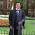if tri-states are involved + your netlist has errors + you're running a cross-simulation (i.e. part of your design is the synthesized netlist and other part is an rtl) then you might see something similar.

I've seen similar things during cross simulation.

Regards,
AR

7.good explanation.
@solosys, if the clock has a weak pull-up, then your 'Z' will be '1', and in this case, there will not be any transition since it's a '1' to '1'. So (clk'event and clk='1') will fire, but again rising_edge(clk) will not fire, because the previous value was 'Z' (and at the board level, it's weakly pulled to '1'). So, rising_edge() and falling_edge() will really check if there is a transition from '0' to '1' (or '1' to '0'), and like vipin said, makes it safe and bug free.

8.But how do these two translate into gate level? Both the coding styles generate a rising/falling edge flip flop.
Is there a type of flip flop which can detect a weak pull up?

1.At gate level, you traditionally, with discrete devices,may use two inverters and a gate(could be xor for a positive pulse). the clock is coupled to the two inverters in series and at the same time to one of the inputs at the xor gate. In real life, the two inverters have more delay than a cable, so for the propagation delay time of two inverters, you will get a pulse from the xor gate. see more about this and how to implement it on google OR this site(quite good) :http://www.twyman.org.uk/clock_recovery/, go down to the edge detection part.

9.Only your simulation is 'bug free' by using rising_edge()/falling_edge(). The SYNTHESIZED logic will be deterministic. Here is a FAIL example where these functions will kick you in the face:
In the case of an input used a a clock: a valid clock edge could also be 'L' to '1' and 'H' to '0'. These would be ignored by the rising_edge() and falling_edge() functions. An example of this would be a wire-or edge triggered interrupt input. So 'glitch free' becomes 'wrong'.

1.This is not true, 'L' to '1' and 'H' to '0' transitions will be picked up by rising_edge() and falling_edge() due to the To_X01() conversion function which maps 0 -> 0, 1 -> 1, H -> 1, L -> 0 and others -> X thus 'L' to '1' and 'H' to '0' transitions will be seen by the functions as '0' to '1' and '1' to '0' transitions.

10.Actually dshawnw, I believe you might be wrong. If you look again at the definition of rising_edge/falling_edge functions, the signal is passed through a "To_X01" conversion table which converts 'H' to '1' and 'L' to '0' before the comparison is evaluated, so the comparisons still hold true for 'L' to '1' and 'H' to '0' transitions just as they do for '1' to '0' and '0' to '1'.

11.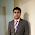its realllllly good, great explaination, thank u for ur help

12.thanks for explaining superbly with an example! :)

13.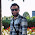thanks for you....

14.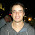Very good explanation. Thank you

15.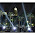It is nice information.